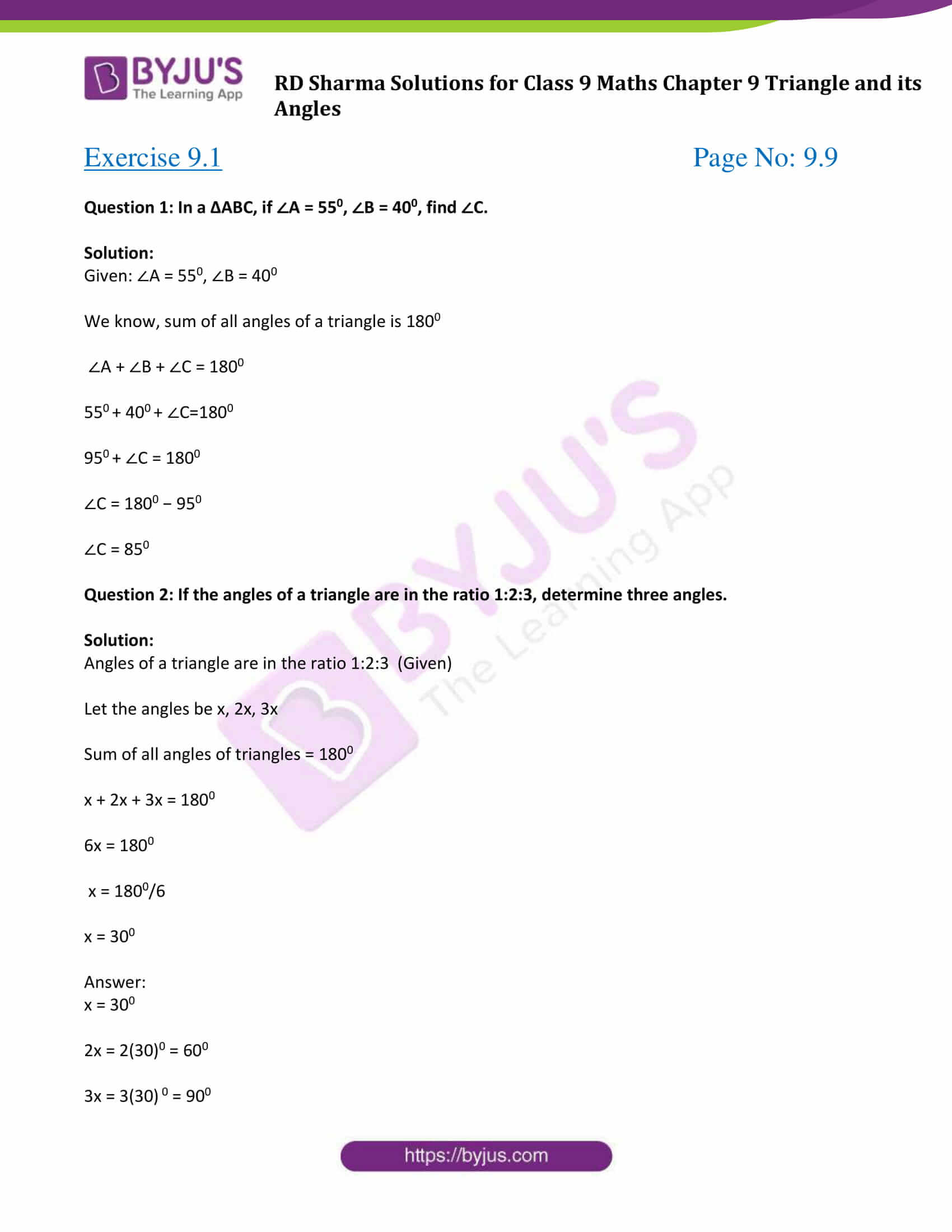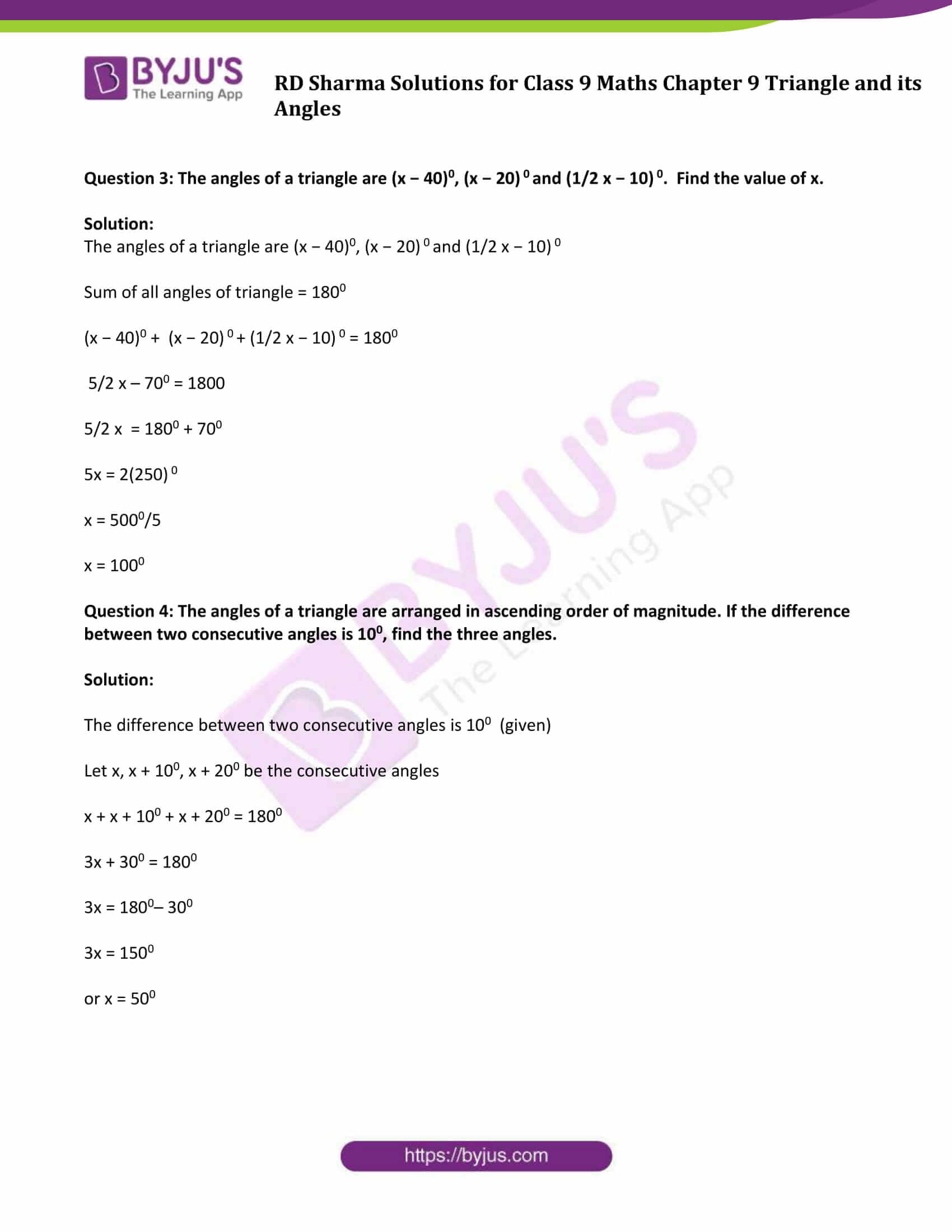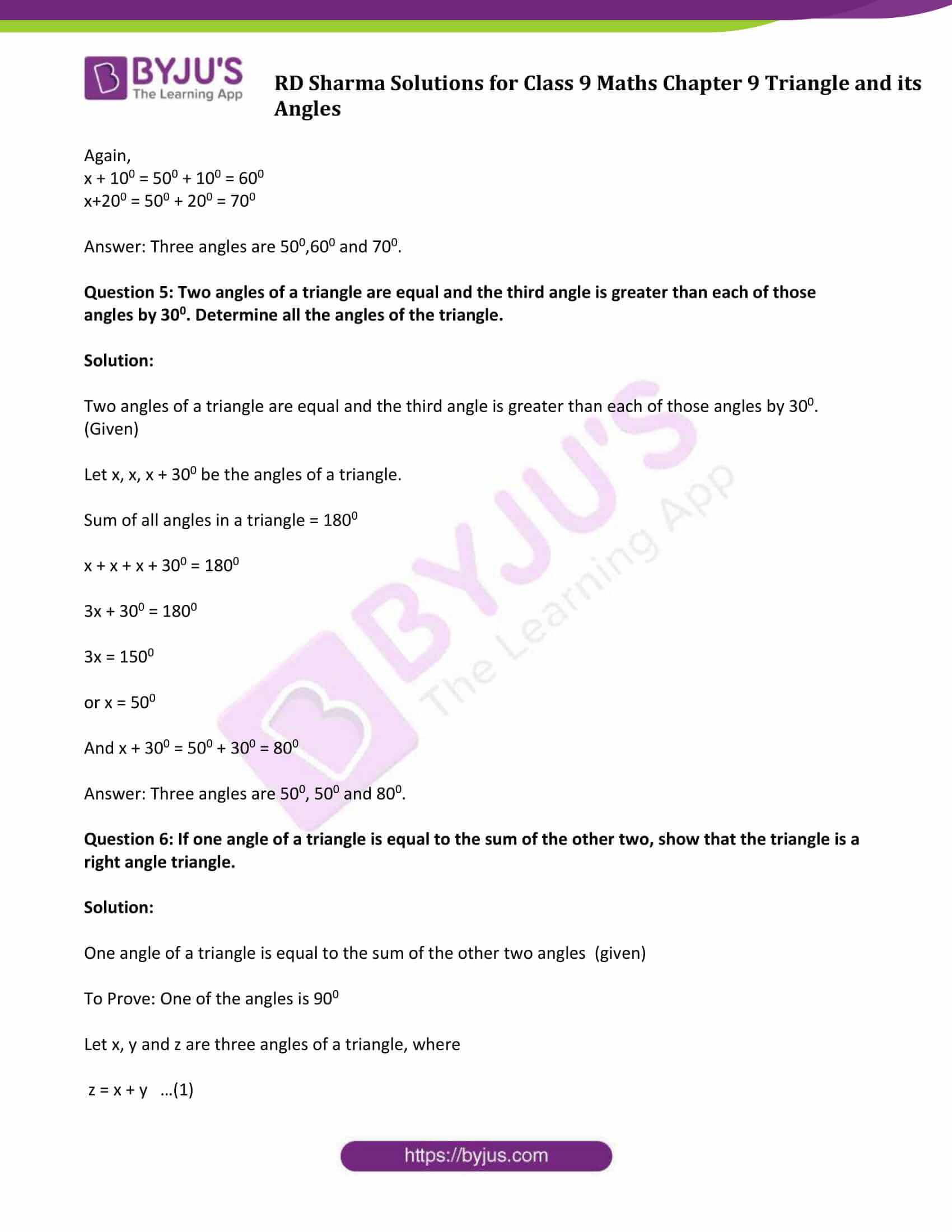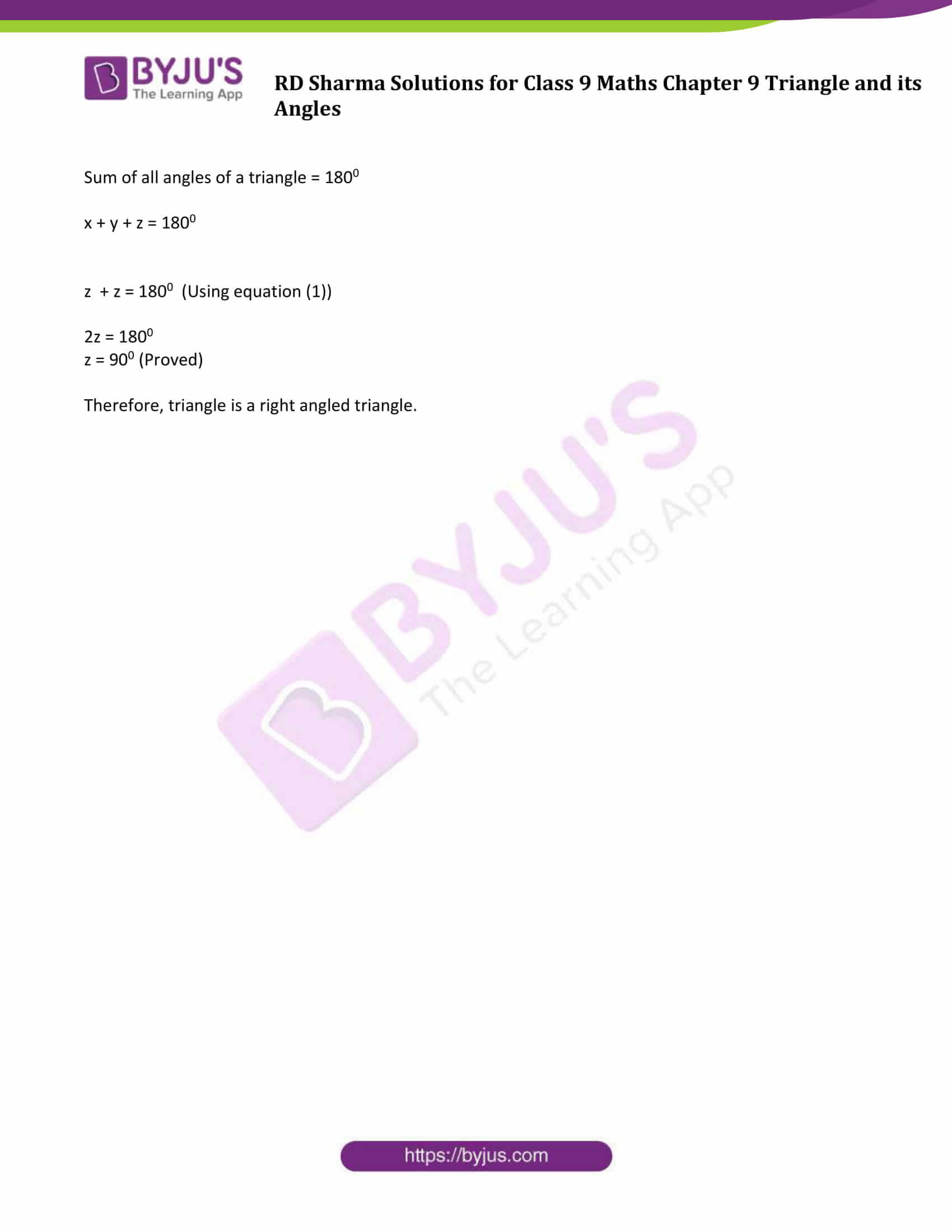# RD Sharma Solutions for Class 9 Maths Chapter 9 Triangle and its Angles Exercise 9.1

RD Sharma Solutions for Class 9 Maths are given here for Chapter 9 – Triangle and its Angles. The RD Sharma solutions that we are offering here have been carefully designed for class 9 students to help them tackle all the difficult Math problems. The Solutions for RD Sharma Class 9 Maths Chapter 9 exercise 9.1 includes solved problems and step by step explanations given by our experts to further helps students find alternative ways to solve a question.

## Download PDF of RD Sharma Solutions for Class 9 Maths Chapter 9 Triangle and its Angles Exercise 9.1### Access Answers to Maths RD Sharma Solutions for Class 9 Chapter 9 Triangle and its Angles Exercise 9.1

Question 1: In a ΔABC, if ∠A = 550, ∠B = 400, find ∠C.

Solution:

Given: ∠A = 550, ∠B = 400

We know, sum of all angles of a triangle is 1800

∠A + ∠B + ∠C = 1800

550 + 400 + ∠C=1800

950 + ∠C = 1800

∠C = 1800 − 950

∠C = 850

Question 2: If the angles of a triangle are in the ratio 1:2:3, determine three angles.

Solution:

Angles of a triangle are in the ratio 1:2:3 (Given)

Let the angles be x, 2x, 3x

Sum of all angles of triangles = 1800

x + 2x + 3x = 1800

6x = 1800

x = 1800/6

x = 300

x = 300

2x = 2(30)0 = 600

3x = 3(30) 0 = 900

Question 3: The angles of a triangle are (x − 40)0, (x − 20) 0 and (1/2 x − 10) 0. Find the value of x.

Solution:

The angles of a triangle are (x − 40)0, (x − 20) 0 and (1/2 x − 10) 0

Sum of all angles of triangle = 1800

(x − 40)0 + (x − 20) 0 + (1/2 x − 10) 0 = 1800

5/2 x – 700 = 1800

5/2 x = 1800 + 700

5x = 2(250) 0

x = 5000/5

x = 1000

Question 4: The angles of a triangle are arranged in ascending order of magnitude. If the difference between two consecutive angles is 100, find the three angles.

Solution:

The difference between two consecutive angles is 100 (given)

Let x, x + 100, x + 200 be the consecutive angles

x + x + 100 + x + 200 = 1800

3x + 300 = 1800

3x = 1800– 300

3x = 1500

or x = 500

Again,

x + 100 = 500 + 100 = 600

x+200 = 500 + 200 = 700

Answer: Three angles are 500,600 and 700.

Question 5: Two angles of a triangle are equal and the third angle is greater than each of those angles by 300. Determine all the angles of the triangle.

Solution:

Two angles of a triangle are equal and the third angle is greater than each of those angles by 300. (Given)

Let x, x, x + 300 be the angles of a triangle.

Sum of all angles in a triangle = 1800

x + x + x + 300 = 1800

3x + 300 = 1800

3x = 1500

or x = 500

And x + 300 = 500 + 300 = 800

Answer: Three angles are 500, 500 and 800.

Question 6: If one angle of a triangle is equal to the sum of the other two, show that the triangle is a right angle triangle.

Solution:

One angle of a triangle is equal to the sum of the other two angles (given)

To Prove: One of the angles is 900

Let x, y and z are three angles of a triangle, where

z = x + y …(1)

Sum of all angles of a triangle = 1800

x + y + z = 1800

z + z = 1800 (Using equation (1))

2z = 1800

z = 900 (Proved)

Therefore, triangle is a right-angled triangle.

## RD Sharma Solutions for Class 9 Maths Chapter 9 Triangle and its Angles Exercise 9.1

RD Sharma Solutions Class 9 Maths Chapter 9 Triangle and its Angles Exercise 9.1 is based on the following topics and subtopics:

• Types of triangles
• – Scalene triangle
• – Isosceles triangle
• – Equilateral triangle
• – Acute Triangle
• – Right Angle and
• – Obtuse Triangle
• Angle sum property of a triangle